# Number of Protons Number of Electrons Number of

• Slides: 14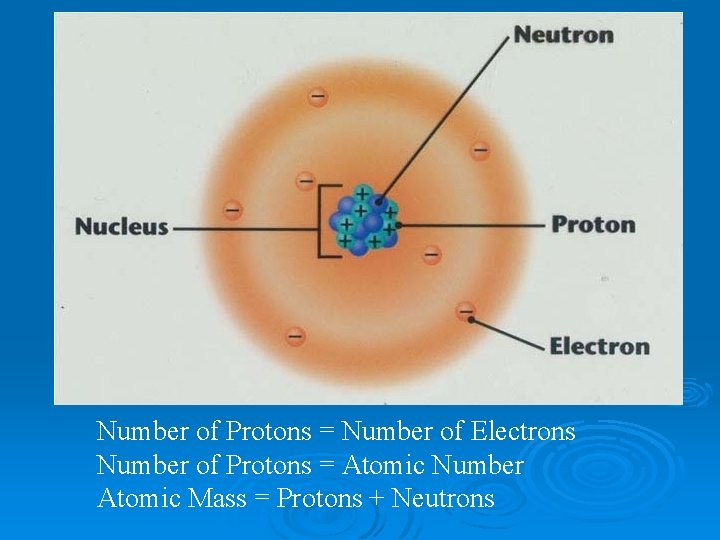Number of Protons = Number of Electrons Number of Protons = Atomic Number Atomic Mass = Protons + Neutrons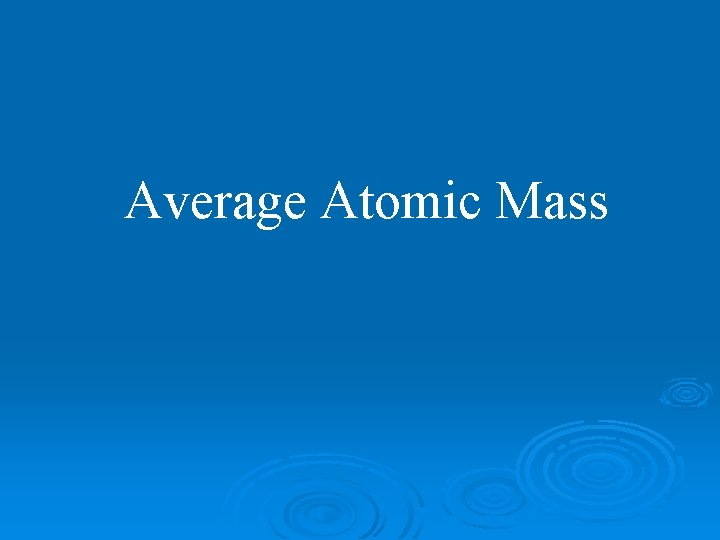Average Atomic MassAverage Atomic Mass The atomic masses reported in the periodic table represent the weighted average of the masses of the naturally occurring isotopes of that elementWeighted Avg. of Test Grades Suppose you have: 1 test of 0% and 9 tests of 90% What if I said your average was 45? (0 + 90 = 90; Divide by 2 = 45) Would you be happy?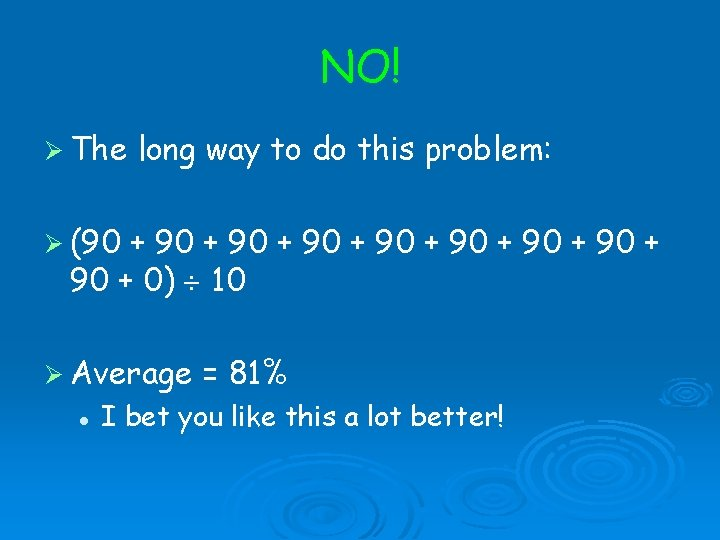NO! The long way to do this problem: (90 + 90 + 90 + 0) 10 Average = 81% I bet you like this a lot better!The smart way to do this problem! grades of 90: 9 out of 10 is 90% grade 0 f 0: 1 out of 10 is 10% So 90% of the test grades are 90 10% of the test grades are 0 (0. 90 X 90) + (0. 10 X 0) = 81 + 0 Average = 81%Weighted average: Smarter way to calculate the average if you have numerous items to average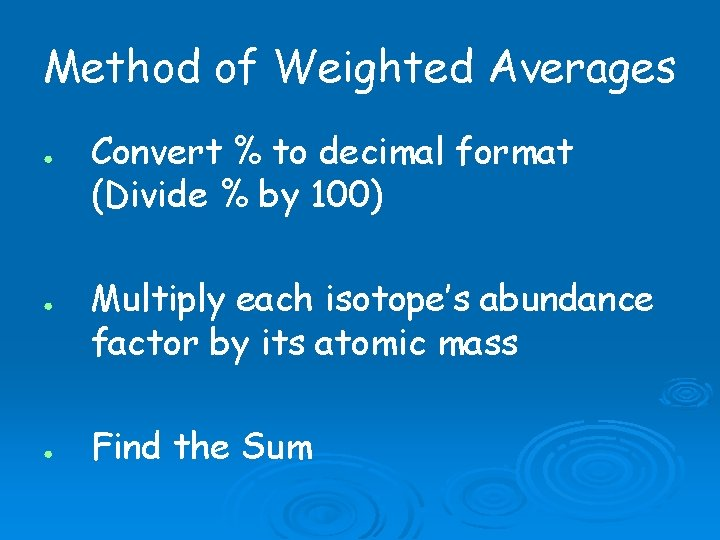Method of Weighted Averages ● ● ● Convert % to decimal format (Divide % by 100) Multiply each isotope’s abundance factor by its atomic mass Find the SumAvg. Atomic Mass of Chlorine has two isotopes. Percent Abundance Mass Isotope 1 75. 770% 34. 969 amu Isotope 2 24. 230% 36. 966 amu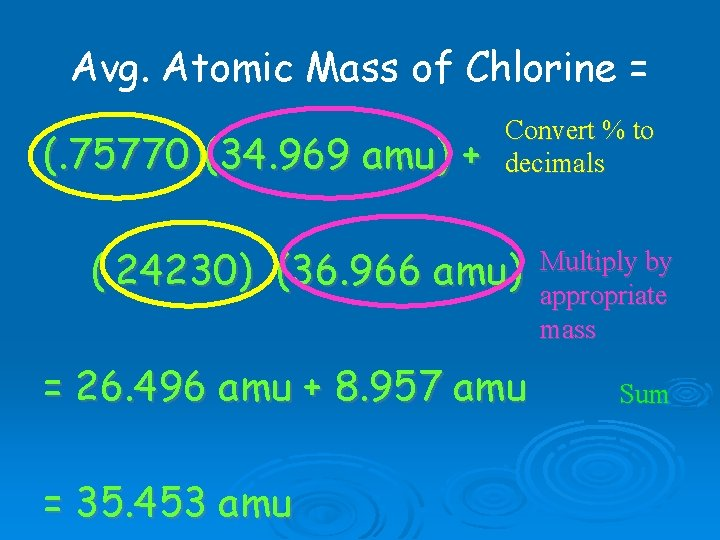Avg. Atomic Mass of Chlorine = (. 75770)(34. 969 amu) + Convert % to decimals (. 24230) (36. 966 amu) = 26. 496 amu + 8. 957 amu = 35. 453 amu Multiply by appropriate mass Sum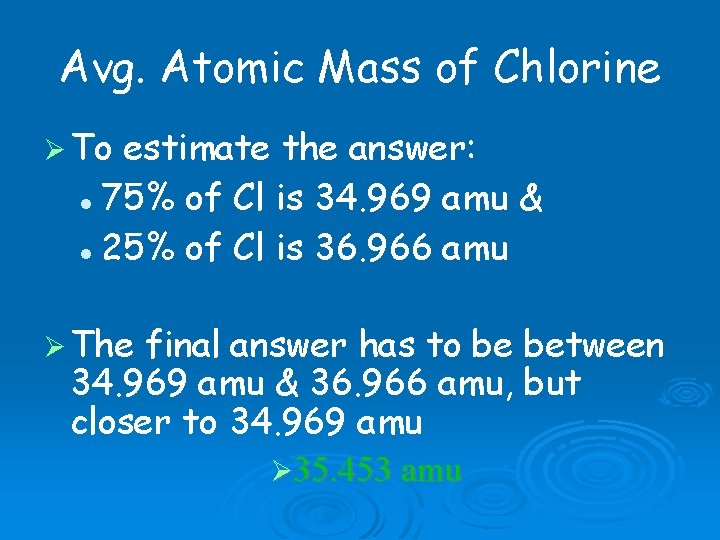Avg. Atomic Mass of Chlorine To estimate the answer: 75% of Cl is 34. 969 amu & 25% of Cl is 36. 966 amu The final answer has to be between 34. 969 amu & 36. 966 amu, but closer to 34. 969 amu 35. 453 amu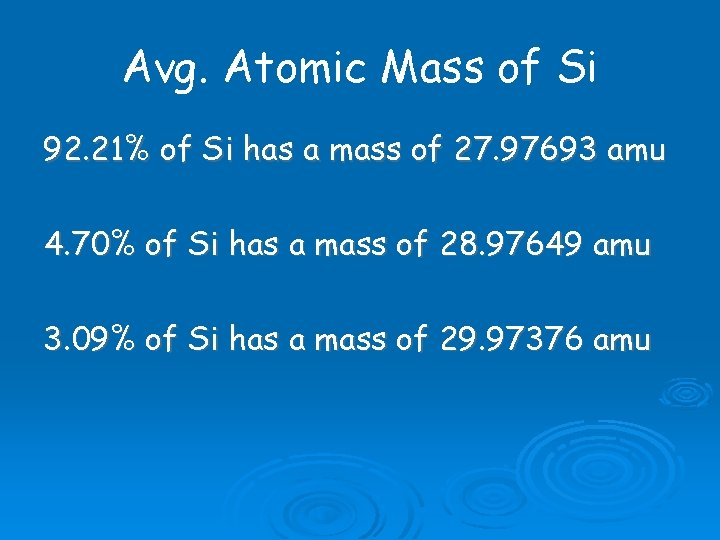Avg. Atomic Mass of Si 92. 21% of Si has a mass of 27. 97693 amu 4. 70% of Si has a mass of 28. 97649 amu 3. 09% of Si has a mass of 29. 97376 amu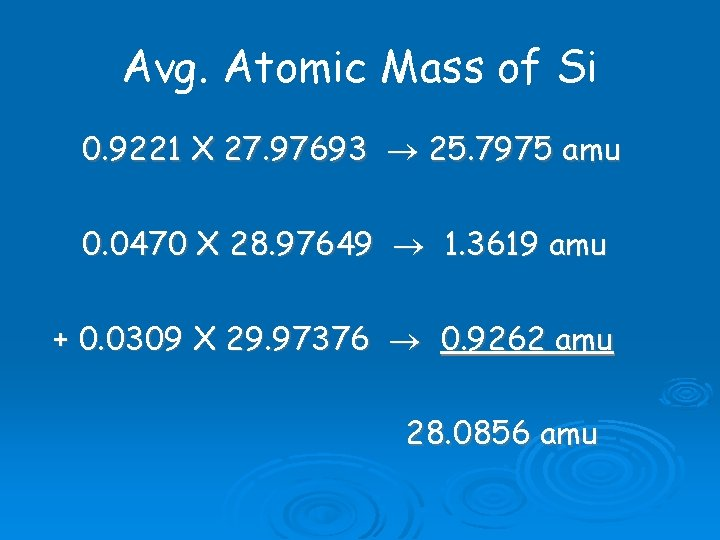Avg. Atomic Mass of Si 0. 9221 X 27. 97693 25. 7975 amu 0. 0470 X 28. 97649 1. 3619 amu + 0. 0309 X 29. 97376 0. 9262 amu 28. 0856 amu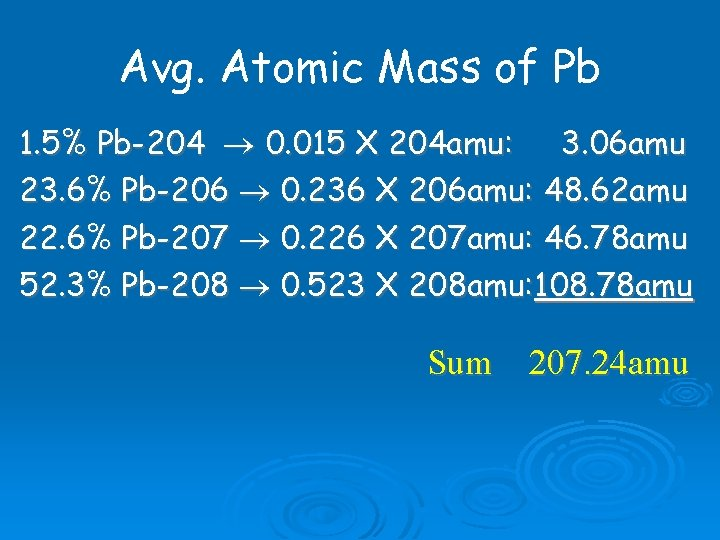Avg. Atomic Mass of Pb 1. 5% Pb-204 0. 015 X 204 amu: 3. 06 amu 23. 6% Pb-206 0. 236 X 206 amu: 48. 62 amu 22. 6% Pb-207 0. 226 X 207 amu: 46. 78 amu 52. 3% Pb-208 0. 523 X 208 amu: 108. 78 amu Sum 207. 24 amu